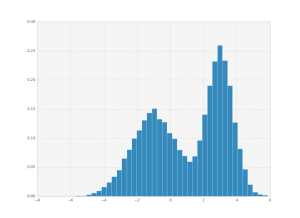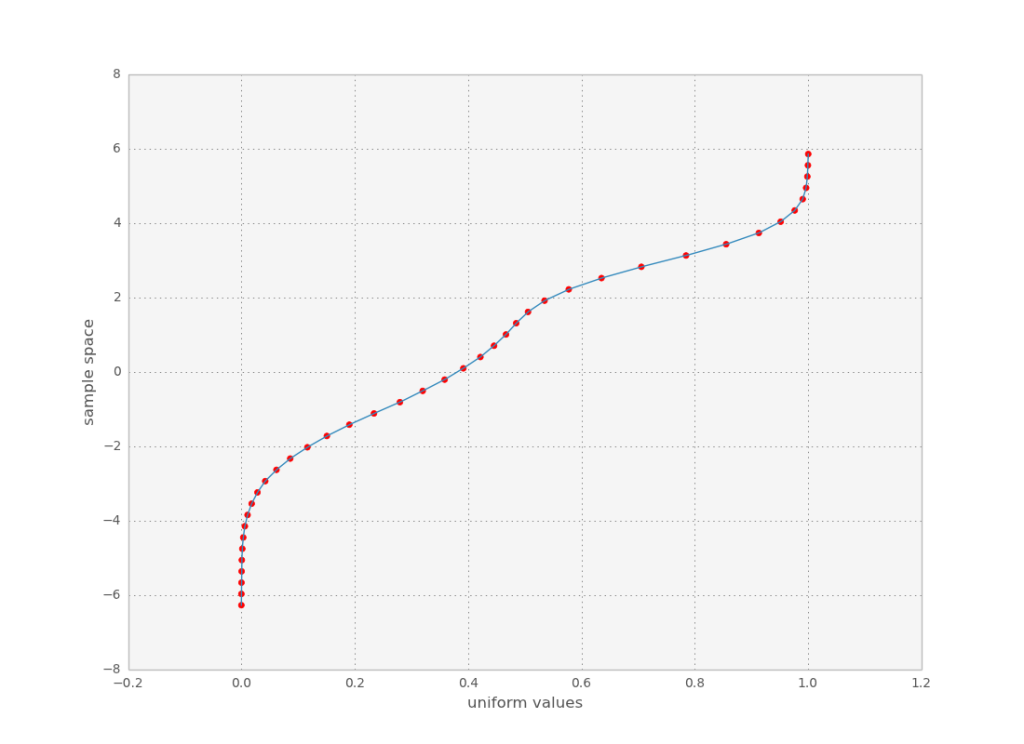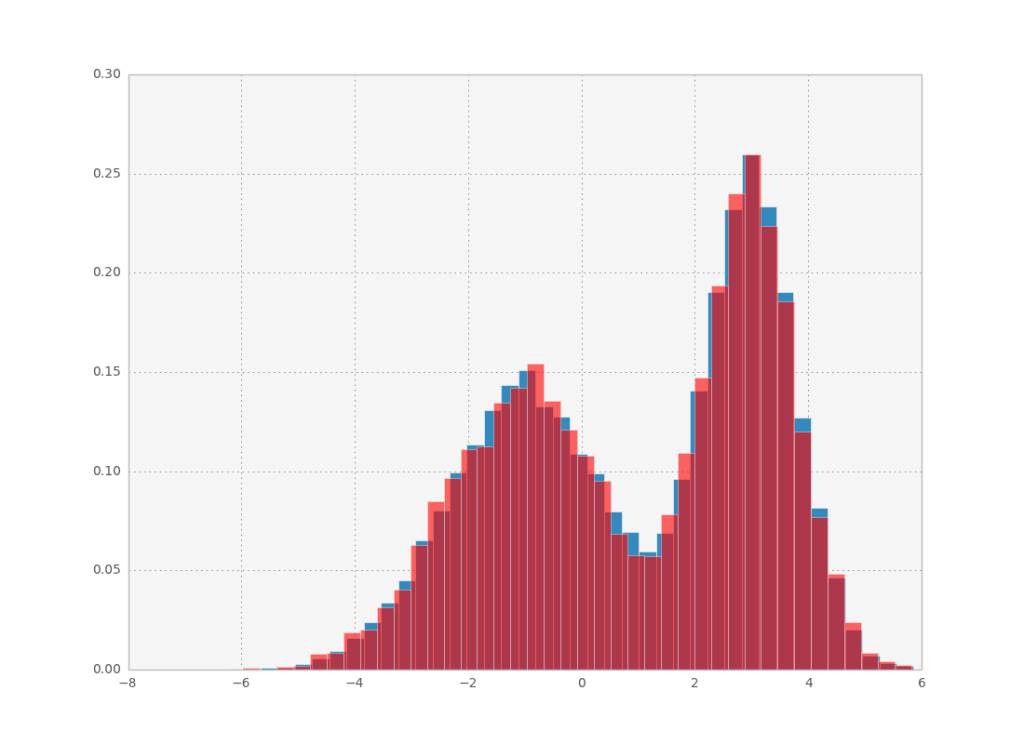# Pilot waves in fluid dynamics

This is so cool. Source

# The link between thermodynamics and inference

In recent blog posts I talked a bit about how many aspects of maximum entropy were analogous to methods in statistical physics. In this short post, I’ll summarize the most interesting similarities. In bayesian inference, we are usually interested in the posterior distribution of some parameters $\theta$ given the data d. This posterior can be written as a boltzmann distribution:

$$P(\theta|d)=\frac{P(\theta,d)}{P(d)}=\left.\frac{e^{-\beta H(\theta,d)}}{Z}\right|_{\beta=1}$$

with $H(\theta,d) = -\log P(\theta,d)/\beta$ and $Z=\int d\theta\;e^{-\beta H(\theta,d)}$. I’ll note that we are working with units such that $k_B=1$ and thus $\beta=1/T$.

The energy is just the expectation value of the hamiltonian H (note that the expectation is taken with respect to $P(\theta|d)$):

$$E = \langle H \rangle = -\frac{\partial \log Z}{\partial \beta}$$

And the entropy is equal to

$$S=-\int d\theta\;P(\theta|d)\log P(\theta|d)=\beta\langle H \rangle - \log Z$$

We can also define the free energy, which is

$$F=E\, - \frac{S}{\beta}=-\frac{\log Z}{\beta}$$

A cool way to approximate Z if we can’t calculate it analytically (we usually can’t calculate it numerically for high dimensional problems because the integrals take a very long time to calculate) is to use laplace’s approximation

$$Z=\int d\theta\;e^{-\beta H(\theta,d)}\simeq\sqrt{\frac{2\pi}{\beta|H'(\theta^*)|}}e^{-\beta H(\theta^*)}$$

where $|H’(\theta^*)|$ is the determinant of the hessian of the hamiltonian (say that 3 times real fast) and $\theta^*$ is such that $H(\theta^*)=\min H(\theta)$ (minimum because of the minus sign). Needless to say this approximation works best for small temperature ($\beta\rightarrow\infty$) which might not be close to the correct value at $\beta=1$. $\theta^*$ is known as the maximum a posteriori (MAP) estimate. Expectation values can also be approximated in a similar way:

$$\langle f(\theta) \rangle = \int d\theta \; f(\theta) P(\theta|d) \simeq\sqrt{\frac{2\pi}{\beta|H'(\theta^*)|}} f(\theta^*)P(\theta^*|d)$$

So the MAP estimate is defined as $\text{argmax}_{\theta} P(\theta|d)$. The result won’t change if we take the log of the posterior, which leads to a form similar to the entropy:

\begin{align}\theta_{\text{MAP}}&=\text{argmax}_{\theta} (-\beta H - \log Z)\\&=\text{argmax}_{\theta} (-2\beta H + S)\end{align}

Funny, huh? For infinite temperature ($\beta=0$) the parameters reflect total lack of knowledge: the entropy is maximized. As we lower the temperature, the energy term contributes more, reflecting the information provided by the data, until at temperature zero we would only care about the data contribution and ignore the entropy term.

(This is also the basic idea for the simulated annealing optimization algorithm, where in that case the objective function plays the role of the energy and the algorithm walks around phase space randomly, with jump size proportional to the temperature. The annealing schedule progressively lowers the temperature, restricting the random walk to regions of high objective function value, until it freezes at some point.)

Another cool connection is the fact that the heat capacity is given by

$$C(\beta)=\beta^2\langle (\Delta H)^2 \rangle=\beta^2\langle (H-\langle H \rangle)^2 \rangle=\beta^2\frac{\partial^2 \log Z}{\partial \beta^2}$$

In the paper I looked at last time, the authors used this fact to estimate the entropy: they calculated $\langle (\Delta H)^2 \rangle$ by MCMC for various betas and used the relation $S = \, \int_{1}^{\infty} d\beta\; \frac{1}{\beta} C(\beta)$

# Review of Searching for Collective Behavior in a Large Network of Sensory Neurons

Last time I reviewed the principle of maximum entropy. Today I am looking at a paper which uses it to create a simplified probabilistic representation of neural dynamics. The idea is to measure the spike trains of each neuron individually (in this case there are around 100 neurons from a salamander retina being measured) and simultaneously. In this way, all correlations in the network are preserved, which allows the construction of a probability distribution describing some features of the network.

Naturally, a probability distribution describing the full network dynamics would need a model of the whole network dynamics, which is not what the authors are aiming at here. Instead, they wish to just capture the correct statistics of the network states. What are the network states? Imagine you bin time into small windows. In each window, each neuron will be spiking or not. Then, for each time point you will have a binary word with 100 bits, where each a 1 corresponds to a spike and a -1 to silence. This is a network state, which we will represent by $\boldsymbol{\sigma}$.

So, the goal is to get $P(\boldsymbol{\sigma})$. It would be more interesting to have something like $P(\boldsymbol{\sigma}_{t+1}|\boldsymbol{\sigma}_t)$ (subscript denoting time) but we don’t always get what we want, now do we? It is a much harder problem to get this conditional probability, so we’ll have to settle for the overall probability of each state. According to maximum entropy, this distribution will be given by

$$P(\boldsymbol{\sigma})=\frac{1}{Z}\exp\left(-\sum_i \lambda_i f_i(\boldsymbol{\sigma})\right)$$

So now it is necessary to define which expectation values will be constrained. The authors have decided to constrain the following:

1. The mean. The function is $f_i=\sigma_i$, with one $i$ for each dimension, and thus there will be a term $-\sum_i h_i \sigma_i$ in the exponential (the $\lambda$ was renamed to $h$ due to thermodynamical reasons, as the first two terms in this model are equivalent to the ising model). 2. Pairwise correlations. This is equivalent to the second order moment, with $f_{ij}=\sigma_i\sigma_j$. The term added to the exponential will be $-1/2\sum_{i,j}J_{ij}\sigma_i\sigma_j$, again with the lagrange multipliers renamed. 3. The proportion of spiking neurons vs. silent ones. To define this, the authors propose a distribution $P(K)=\sum_{\boldsymbol{\sigma}}\, P(\boldsymbol{\sigma})\,\delta(\sum_i \sigma_i, 2K-N)$ (because K spins will cancel out K other spins, you’re left with $N-2K$ spins). This distribution is fixed by its N moments, which reduce to

$$\langle K^k\rangle=\sum_{\boldsymbol{\sigma}} \left(\sum_i \sigma_i\right)^k P(\boldsymbol{\sigma})$$

once you kill the delta. It is now clear that the max entropy function will be $f_k=\left(\sum_i \sigma_i\right)^k$ corresponding to a term

$$-\sum_k^n \lambda_k \left(\sum_i \sigma_i\right)^k$$

in the exponential (no renaming convention here). I am a bit suspicious of this term here since the different powers of $\sum_i \sigma_i$ are not really independent quantities, which might mean that the model is being overfit. I would be interested in seeing a comparison between this term and a simpler one, with just a single term ($k=1$) considered.

So their probability distribution looks like

$$P(\boldsymbol{\sigma})\propto\exp\left(-\sum_i h_i \sigma_i-1/2\sum_{i,j}J_{ij}\sigma_i\sigma_j-\sum_k^n \lambda_k \left(\sum_i \sigma_i\right)^k\right)$$

which is kind of the ising model with the addition of that last term. The crucial step is now to find ${\mathbf{h}, \mathbf{J},\boldsymbol{\lambda}}$ such that the expectations of the distribution match the ones calculated from the data. So one can run an optimization algorithm which will change the values of those parameters until the expectations for the distribution match (or are close enough to) the experimental ones.

But $P(\boldsymbol{\sigma})$ as defined has no analytic expression for the desired expectation values,  so they must be calculated numerically. The authors use MCMC to calculate them, because of the high dimensionality of the problem. Of course, this is super computationally expensive, so they use a little trick to make it go faster. The idea is that if you only change the parameters slightly, the distribution will also only change slightly, and therefore the MCMC samples can be reused. Consider a max ent distribution with a vector of parameters $\lambda$. You can rewrite the expectation value of a function g as

\begin{aligned}\langle g\rangle_{\lambda'}&=\sum_{\boldsymbol{\sigma}} g(\boldsymbol{\sigma}) P_{\lambda'}(\boldsymbol{\sigma})\\&=\sum_{\boldsymbol{\sigma}} g(\boldsymbol{\sigma}) \frac{P_{\lambda'}(\boldsymbol{\sigma})}{P_{\lambda}(\boldsymbol{\sigma})}P_{\lambda}(\boldsymbol{\sigma})\\&=\sum_{\boldsymbol{\sigma}}  g(\boldsymbol{\sigma}) \frac{Z_{\lambda}}{Z_{\lambda'}} \exp\left(-(\lambda'-\lambda).f(\boldsymbol{\sigma})\right) P_{\lambda}(\boldsymbol{\sigma})\\&=\frac{Z_{\lambda}}{Z_{\lambda'}}  \left\langle g \exp\left(-(\lambda'-\lambda).f\right) \right\rangle_{\lambda}\\&=\frac{\left\langle g \exp\left(-(\lambda'-\lambda).f\right) \right\rangle_{\lambda}}{\left\langle \exp\left(-(\lambda'-\lambda).f\right) \right\rangle_{\lambda}} \end{aligned}

So if you have the samples for the parameter set $\lambda$ you can estimate the value for the parameter set $\lambda’$ with little error (indeed, the above formula is exact, any error comes from the fact that we have finite samples from MC). After you moved too far away from the distribution, your samples will probably not do a very good job of approximating the distribution, so the authors propose resampling after a given number of evaluations (but they don’t mention how they chose the max number of evaluations, this is something that probably could use a bit more discussion). Okay, so now they just plug this in their favorite (derivative-free) optimizer and they’re golden.

They then go on to discuss the results, where they show that this procedure produces a pretty good fit to the data with apparently low overfitting. I’d invite you to read the full paper, which has a lot of discussion on the actual neuroscience and information theory.

# Maximum entropy: a primer and some recent applications

I’ll let Caticha summarize the principle of maximum entropy:

Among all possible probability distributions that agree with whatever we know select that particular distribution that reflects maximum ignorance about everything else. Since ignorance is measured by entropy, the method is mathematically implemented by selecting the distribution that maximizes entropy subject to the constraints imposed by the available information.

It appears to have been introduced by Jaynes in 57, and has seen a resurgence in the past decade with people taking bayesian inference more seriously. (As an aside, Jayne’s posthumously published book is well worth a read, in spite of some cringeworthy rants peppered throughout.) I won’t dwell too much on the philosophy as the two previously mentioned sources have already gone into great detail to justify the method.

Usually we consider constraints which are linear in the probabilities, namely we constrain the probability distribution to have specific expectation values. Consider that we know the expectation values of a certain set of functions $f^k$. Then, $p(x)$ should be such that

$$\langle f^k \rangle = \int dx \; p(x) f^k(x)$$

for all k. Let’s omit the notation $(x)$ for simplicity. Then, we can use variational calculus to find p which minimizes the functional

$$S[p]\; - \alpha \int dx\; p\; - \sum_k \lambda_k \langle f^k \rangle$$

The constraint with $\alpha$ is the normalization condition and $S$ is the shannon entropy.

The solution to this is

$$p = \frac{1}{Z}\exp\left(-\sum_k\lambda_k f^k \right)$$

with

$$Z=\int dx \; \exp \left(-\sum_k\lambda_k f^k \right)$$

the partition function (which is just the normalization constant). Now, we can find the remaining multipliers by solving the system of equations

$$-\frac{\partial \log Z}{\partial \lambda_k} = \langle f^k \rangle$$

I’ll let you confirm that if we fix the mean and variance we get a gaussian distribution. Go on, I’ll wait.

## Power law distributions with maxent

I read a recent paper on PNAS which shows how to use this formalism to derive an intuition on why distributions with power law tails are so ubiquitous. It is not very well written, but I think I successfully deduced what the authors meant. Suppose you have a system with a given number of particles, where you ‘pay’ a fixed price for each particle to join. If you consider the first one to already be there, the total paid cost is $f(k)=(k-1)\mu$, with mu the chemical potential (now we are talking about discrete states, now the k indexes each state, or rather the number of particles in the cluster).

By bundling every constant into a $\mu^{\circ}$ factor, its not necessary to specify the actual value of the expectation and determine the lagrange multiplier: whatever it is, the distribution will look like $p_k=\frac{\exp(-\mu^{\circ} k)}{Z}$ with this arbitrary $\mu’$ factor (remember Z is just a normalization constant). This is an exponential distribution, which means events far away from the mean are comparatively very rare. The authors now propose to look at economies of scale - what if it gets cheaper to add a new particle as the cluster grows? Then, the cost is $k_0\mu/(k+k_0)$, which is a hill type function and where $k_0$ describes how much the cost is spread out with each additional particle. So the function f becomes $f(k)=\sum_{j=1}^{k-1} k_0\mu/(j+k_0)$ . Then you repeat the calculation and you get

$$p_k=\frac{\exp(-\mu^{\circ} k_0 \Psi(k+k_0))}{Z}$$

Here, $\Psi$ is the digamma function and it’s just a fancy way of hiding the sum you saw in the function $f$ (when you do that, you also get a constant term which then cancels out). You can expand it for large $k$ and get $\Psi \sim \log(k+k_0-1/2)$. Then the probability distribution is $p_k \sim \left(k+k_0-1/2\right)^{-\mu^{\circ} k_0}$. Cool. The authors tested this by fitting $\mu^{\circ}$ and $k_0$ to various datasets with power law distributions, which doesn’t really show much more since we already know they are power laws, and the expression they fit is a power law. The main message here is that you can get a power law from the maximum entropy principle, which suggests a sort of universality among systems brought about by this kind of cost amortization.

In the next post, I’ll talk about a more complicated application of the maximum entropy principle to neural codes.

# How to do inverse transformation sampling in scipy and numpy

Let’s say you have some data which follows a certain probability distribution. You can create a histogram and visualize the probability distribution, but now you want to sample from it. How do you go about doing this with python?The short answer:

import numpy as np
import scipy.interpolate as interpolate

def inverse_transform_sampling(data, n_bins=40, n_samples=1000):
hist, bin_edges = np.histogram(data, bins=n_bins, density=True)
cum_values = np.zeros(bin_edges.shape)
cum_values[1:] = np.cumsum(hist*np.diff(bin_edges))
inv_cdf = interpolate.interp1d(cum_values, bin_edges)
r = np.random.rand(n_samples)
return inv_cdf(r)


The long answer:

You do inverse transform sampling, which is just a method to rescale a uniform random variable to have the probability distribution we want. The idea is that the cumulative distribution function for the histogram you have maps the random variable’s space of possible values to the region [0,1]. If you invert it, you can sample uniform random numbers and transform them to your target distribution!To implement this, we calculate the CDF for each bin in the histogram (red points above) and interpolate it using scipy’s interpolate functions. Then we just need to sample uniform random points and pass them through the inverse CDF! Here is how it looks: